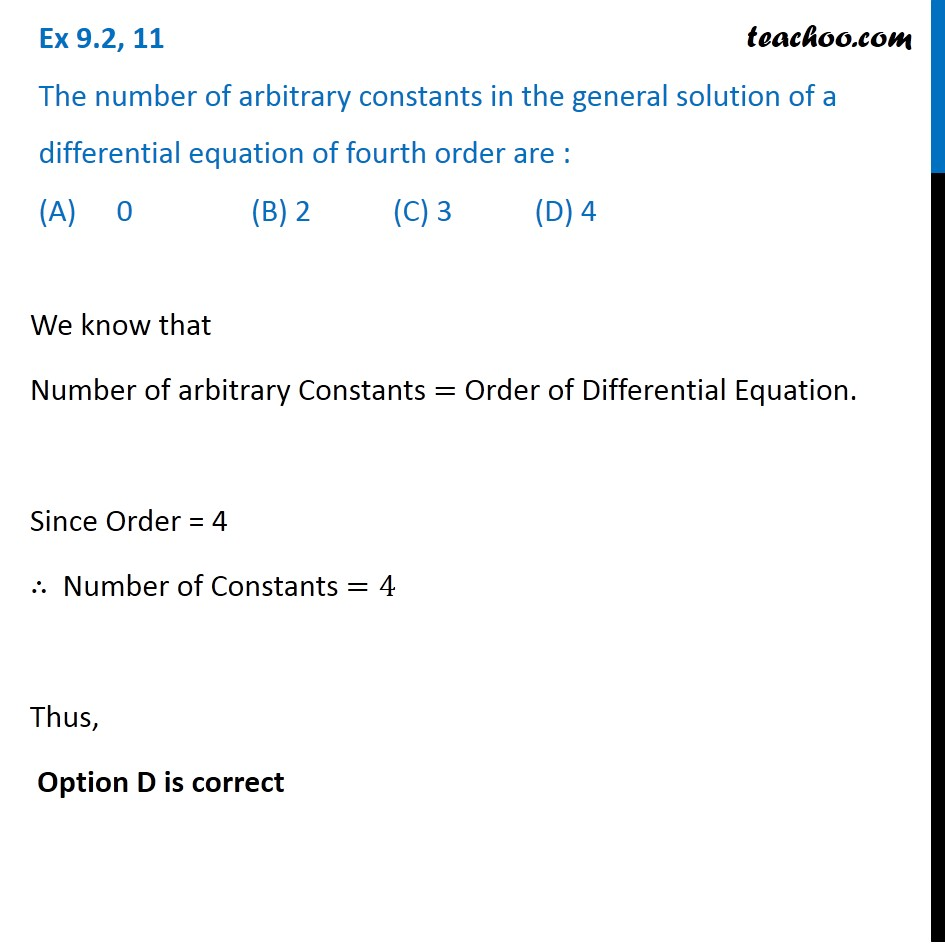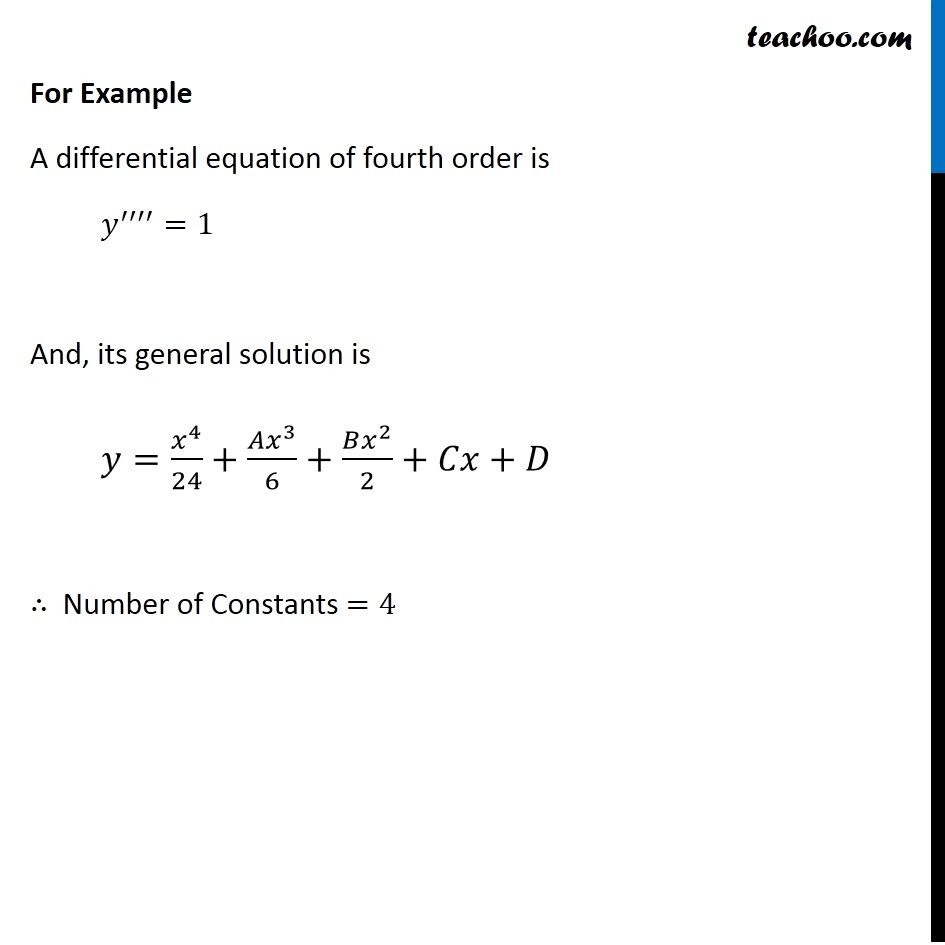Gen and Particular Solution

Chapter 9 Class 12 Differential Equations
Concept wiseIntroducing your new favourite teacher - Teachoo Black, at only ₹83 per month

### Transcript

Ex 9.2, 11 The number of arbitrary constants in the general solution of a differential equation of fourth order are : (A) 0 (B) 2 (C) 3 (D) 4 We know that Number of arbitrary Constants = Order of Differential Equation. Since Order = 4 ∴ Number of Constants =4 Thus, Option D is correct For Example A differential equation of fourth order is 𝑦^′′′′=1 And, its general solution is 𝑦=𝑥^4/24+(𝐴𝑥^3)/6+(𝐵𝑥^2)/2+𝐶𝑥+𝐷 ∴ Number of Constants =4